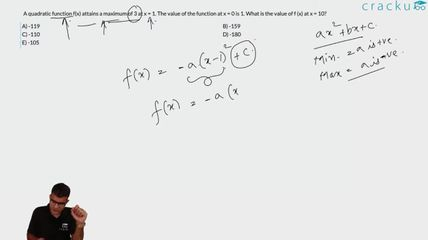Question 25

# A quadratic function f(x) attains a maximum of 3 at x = 1. The value of the function at x = 0 is 1. What is the value of f (x) at x = 10?

Solution

Let the function be $$ax^2 + bx + c$$.

We know that x=0 value is 1 so c=1.

So equation is $$ax^2 + bx + 1$$.

Now max value is 3 at x = 1.

So after substituting we get a + b = 2.

If f(x) attains a maximum at 'a' then the differential of f(x) at x=a, that is, f'(a)=0.

So in this question f'(1)=0

=> 2*(1)*a+b = 0

=> 2a+b = 0.

Solving the equations we get a=-2 and b=4.

$$-2x^2 + 4x + 1$$ is the equation and on substituting x=10, we get -159.

### View Video Solution